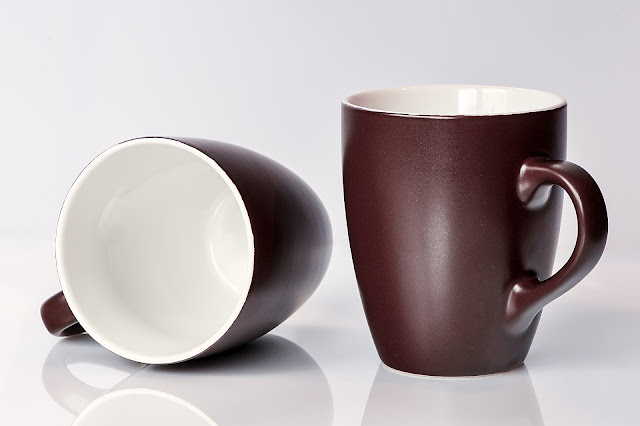# How Many Grams of Water in a Cup:

## How Many Grams of Water in a Cup

At some point, you might find yourself needing to know how many grams of water are in a cup. Whether you're trying out a new recipe, measuring your daily water intake, or simply curious, having accurate information is important. In this article, we'll explore everything you need to know about how many grams of water are in a cup, and more.How Many Grams of Water in a Cup

## Cups to Grams: The Basics

The amount of water in a cup can vary depending on where you are in the world. In the United States, a cup is equal to 8 fluid ounces or approximately 236.6 milliliters. In the United Kingdom and other Commonwealth countries, a cup is equal to 250 milliliters. However, when it comes to converting cups to grams, the weight of the water is what matters most.

In general, one cup of water weighs 240 grams or 8.345 ounces. This means that if you need to convert cups to grams, you can simply multiply the number of cups by 240 to get the total number of grams. For example, if a recipe calls for 2 cups of water, you would need 480 grams of water.

## Factors that Affect Water Weight

While the standard weight of one cup of water is 240 grams, it's important to note that this can vary slightly depending on a few factors. For example, the temperature of the water can affect its weight. Warmer water is less dense than colder water, so a cup of warm water will weigh slightly less than a cup of cold water.

Additionally, the altitude at which you are measuring the water can also impact its weight. At higher altitudes, there is less atmospheric pressure, which can cause water to boil at a lower temperature. This can result in more water being lost as steam, which means that a cup of water measured at a high altitude may weigh slightly less than a cup of water measured at sea level.

## Measuring Water by Weight

When it comes to measuring ingredients accurately, measuring by weight is often the most reliable method. Using a kitchen scale to measure water in grams can help ensure that your recipes turn out consistently every time. Additionally, measuring water by weight is often more precise than using measuring cups, which can vary slightly in size and shape.

To measure water by weight, simply place your measuring cup or container on a kitchen scale and zero it out. Then, slowly add water until you reach the desired weight in grams. This method can be particularly useful when making bread, where precise measurements are essential for the dough to rise properly.

## Conclusion

In conclusion, knowing how many grams of water are in a cup is an important piece of information for anyone who spends time in the kitchen. While the standard weight of one cup of water is 240 grams, it's important to keep in mind that factors like temperature and altitude can cause slight variations in weight. Measuring water by weight using a kitchen scale can help ensure accurate and consistent results in your recipes.

No Comment